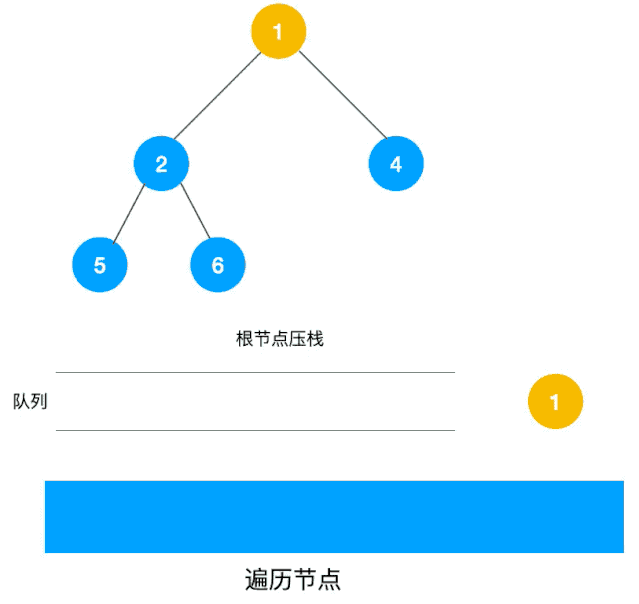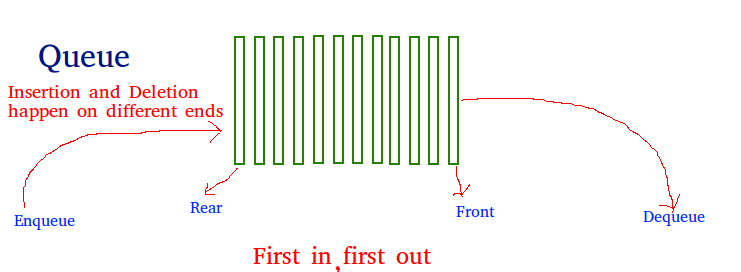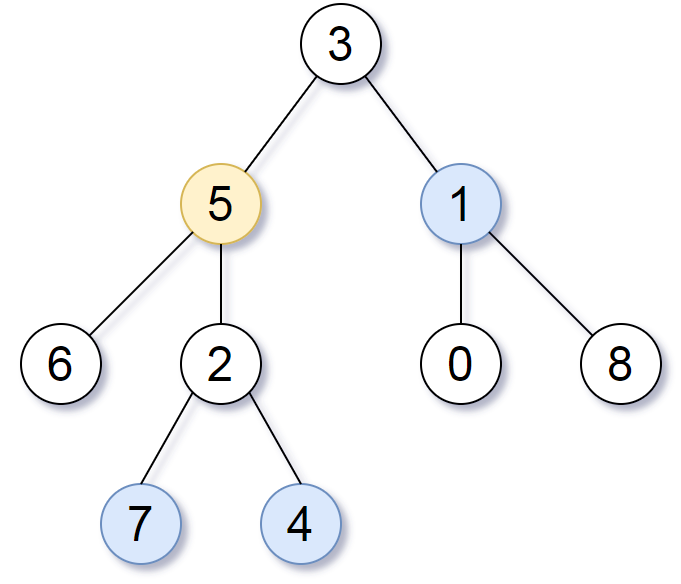## 引子

    3
/ \
9  20
/  \
15   7


### 构建二叉树

void ConstructBinTree(vector<int> &nodes, TreeNode *root)
{
if (nodes.size() == 0 || nodes == INT_MAX)
{
root = nullptr;
return;
}
queue<TreeNode *> iq;
int id = 0;
root->val = nodes[id++];
iq.emplace(root);

while (!iq.empty() && id < nodes.size())
{
TreeNode *node = iq.front();
iq.pop();

// check vectors for left node
if (nodes[id] != INT_MAX)
{
TreeNode *leftNode = new TreeNode;
leftNode->val = nodes[id];
node->left = leftNode;
iq.emplace(leftNode);
}
else
{
node->left = nullptr;
}
id++;

if (nodes[id] != INT_MAX)
{
TreeNode *rightNode = new TreeNode;
rightNode->val = nodes[id];
node->right = rightNode;
iq.emplace(rightNode);
}
else
{
node->right = nullptr;
}
id++;
}
return;
}


void ScanBinMiddle(TreeNode *tree)
{
if (tree == nullptr)
{
return;
}

// handle value
cout << tree->val << " ";

if (tree->left)
{
ScanBinMiddle(tree->left);
}

if (tree->right)
{
ScanBinMiddle(tree->right);
}
}


### 递归解法

#### 描述变量

maxdepth[node]表示以node为根节点的树的最大深度

#### 终止条件

int maxDepth(TreeNode* root)
{
// 终止条件
if (root == nullptr) {
return 0;
}
// 关系式
return max(maxDepth(root->left), maxDepth(root->right)) + 1;
}


### 广度优先搜索算法（BFS）

1. 访问第一层的根节点，如果非空则maxdepth++，否则返回；
2. 查看第一层的根节点是否有左右子树，有的话再分别访问左子树和右子树，maxdepth++
3. 循环步骤2，直到所有的节点都被访问到。1. 我们一次将一层的节点放入到队列中；
2. 判断当前队列是否为空。
• 如果当前的队列不为空，那么将队列中的每个节点pop出来之后再考察这个节点的左右子树，如果有就将它们插入到队列的尾巴，depth++
• 如果当前队列为空，return

int maxDepth(TreeNode* root)
{
// 如果为空，那么返回0
if (root == nullptr) {
return 0;
}

queue<TreeNode *> qu;
int depth = 0;
// 添加根节点
qu.push(root);
while (!qu.empty()) {
int qz = qu.size();
for (int i = 0;i < qz;i++) { // 考察队列中的每个节点，是否有左子树和右子树
TreeNode * node = qu.front();
qu.pop();
// 如果有左右子节点，那么添加到队列中
if (node->left) {
qu.push(node->left);
}

if (node->right) {
qu.push(node->right);
}
}
depth++;
}

return depth;
}## 数据结构

### 队列• 队列头（front），表示队列最开始的元素；
• 队列尾（rear），表示队列最后加入的元素；
• 队列长度，当前的队列长度，就是rear - front + 1；
• 出队（pop），队列头弹出，队列长度-1，front++；
• 入队（push），队列尾添加元素，队列长度+1，rear++

### STL中的queue

C++的stl使用queue表示队列，常用的操作和属性如下表所示

• front()：返回 queue 中第一个元素的引用。如果 queue 是常量，就返回一个常引用；如果 queue 为空，返回值是未定义的。
• back()：返回 queue 中最后一个元素的引用。如果 queue 是常量，就返回一个常引用；如果 queue 为空，返回值是未定义的。
• push(const T& obj)：在 queue 的尾部添加一个元素的副本。这是通过调用底层容器的成员函数 push_back() 来完成的。
• push(T&& obj)：以移动的方式在 queue 的尾部添加元素。这是通过调用底层容器的具有右值引用参数的成员函数 push_back() 来完成的。
• pop()：删除 queue 中的第一个元素。
• size()：返回 queue 中元素的个数。
• empty()：如果 queue 中没有元素的话，返回 true。
• emplace()：用传给 emplace() 的参数调用 T 的构造函数，在 queue 的尾部生成对象。
• swap(queue<T> &other_q)：将当前 queue 中的元素和参数 queue 中的元素交换。它们需要包含相同类型的元素。也可以调用全局函数模板 swap() 来完成同样的操作。

// CPP program to illustrate
// Application of push() and pop() function
#include <iostream>
#include <queue>
using namespace std;

int main()
{
int c = 0;
// Empty Queue
queue<int> myqueue;
myqueue.push(5);
myqueue.push(13);
myqueue.push(0);
myqueue.push(9);
myqueue.push(4);
// queue becomes 5, 13, 0, 9, 4

// Counting number of elements in queue
while (!myqueue.empty()) {
myqueue.pop();
c++;
}
cout << c;
}


## 典型题目

### 二叉树的右视图

vector<int> rightSideView(TreeNode* root)
{
vector<int> rlt;
// empty rlt for empty tree
if (root == nullptr) {
return rlt;
}

queue<TreeNode *> qu;
qu.push(root);
while (!qu.empty()) {
int qz = qu.size();
// add the last node in the current queue
rlt.emplace_back(qu.back()->val);
// add nodes of next layer into the queue
for (int i = 0;i < qz;i++) {
TreeNode * node = qu.front();
qu.pop();
if (node->left) {
qu.push(node->left);
}

if (node->right) {
qu.push(node->right);
}
}
}

return rlt;
}


### 二叉树中所有距离为 K 的结点1. 记录每个节点的father节点，left节点和right节点，将二叉树变成图，表示A与这3个节点的任意一个都是连通的；
2. 初始化目标节点的depth = 1，以该节点为圆心，遍历所有的节点，打印出来所有depth = K + 1的节点

vector<int> distanceK(TreeNode *root, TreeNode *target, int K)
{
unordered_map<TreeNode *, TreeNode *> umap;
vector<int> rlt;

if (root == nullptr || target == nullptr)
{
return rlt;
}

/************ PART 1 ************/
// find the father node of all the nodes in the tree
queue<TreeNode *> qu;
umap[root] = nullptr;
qu.push(root);
while (!qu.empty())
{
int qz = qu.size();
for (int i = 0; i < qz; i++)
{
TreeNode *node = qu.front();
qu.pop();
if (node->left)
{
qu.push(node->left);
// father node
umap[node->left] = node;
}

if (node->right)
{
qu.push(node->right);
// father node
umap[node->right] = node;
}
}
}

/************ PART 2 ************/
// find the node with depth of K
queue<TreeNode *> newQ;
int depth = 0;
unordered_map<TreeNode *, bool> usedmap;
usedmap[target] = true;
newQ.emplace(target);
while (!newQ.empty() && depth <= K)
{
int qz = newQ.size();
for (int i = 0; i < qz; i++)
{
TreeNode *node = newQ.front();
usedmap[node] = true;
newQ.pop();
if (depth == K)
{
rlt.emplace_back(node->val);
continue;
}
if (node->left && (usedmap.count(node->left) == 0))
{
newQ.push(node->left);
}

if (node->right && (usedmap.count(node->right) == 0))
{
newQ.push(node->right);
}

if (umap[node] != nullptr && (usedmap.count(umap[node]) == 0))
{
newQ.push(umap[node]);
}
}
depth++;
}

//BFS for final rlt
return rlt;
}


1. 第一部分遍历二叉树的每一个节点，记录每个节点的父节点，这里我们使用了哈希表来保存每个节点和它的父节点；
2. 第二部分就是核心代码，以target为圆心，将二叉树当作图来遍历，如果这个node有左右节点或者父节点，则表示它跟其他的节点之间联通，则使用BFS算法访问整个图网络。这里尤其要注意，遍历图需要标记当前图中的节点是否被访问过，否则会被多次重复遍历而陷入到死循环中，在这个程序里面，使用usedmap来做这件事，其实也可以使用vector<TreeNode *>来记录。

TreeNode *FindTargetNode(TreeNode *root, int targetVal)
{
if (root == nullptr)
{
return nullptr;
}

queue<TreeNode *> qu;
qu.push(root);
while (!qu.empty())
{
int qz = qu.size();
for (int i = 0; i < qz; i++)
{
TreeNode *node = qu.front();
qu.pop();
if (node->val == targetVal)
{
return node;
}

if (node->left)
{
qu.push(node->left);
}

if (node->right)
{
qu.push(node->right);
}
}
}
}


### 颜色交替的最短路径

class Solution {
enum color {RED, BLUE};
public:
vector<int> shortestAlternatingPaths(int n, vector<vector<int>>& red_edges, vector<vector<int>>& blue_edges) {

// 由于存在自环或者平行边，所以定义哈希表保存每个结点对应的多条边并初始化
unordered_map<int, vector<int>> redGraph;
unordered_map<int, vector<int>> blueGraph;
for (auto& red : red_edges) redGraph[red].push_back(red);
for (auto& blue : blue_edges) blueGraph[blue].push_back(blue);

const int colorNum = 2;
const int maxNode = 100;

// 由于存在环和平行边，用数组 visit[x][y][color]=true 代表从节点x到节点y的且颜色为color的边被访问过，防止重复访问
// 第三维有两维，第0维代表红色是否访问，第1维代表蓝色是否访问
// 所有的点初始化为0代表为被访问过
bool visited[maxNode][maxNode][colorNum];
memset(visited, false, sizeof(visited));

// step用于记录当前的步长，即从节点0到各节点的步长，从0逐渐+1自增
// res代表节点 0 到节点 X 的最短路径的长度，初始化为最大值
int step = 0;
vector<int> res(n, INT_MAX);

// 定义队列进行BFS，并进行初始化，pair<int, int>的意思是 <当前节点, 路径上颜色>
// 队列初始化先进<0, 1>, 再进<0, 0>，即我们先访问蓝色，再访问红色。
queue<pair<int, color>> myQue; // <node, color> means start from node and select the edge with color
myQue.push(make_pair(0, BLUE));
myQue.push(make_pair(0, RED));

while (!myQue.empty())
{
int size = myQue.size();
++step;

for (int i = 0; i < size; i++)
{
// 队首元素出队列，得到其节点，以及颜色
int curNode = myQue.front().first;
int curColor = myQue.front().second;
myQue.pop();

//若当前已访问的为蓝色边，希望下一个节点的边是红色；反之亦然
if (curColor == BLUE)
{
// 遍历当前节点每一个相邻的节点，寻找相连的红色边
for (auto& nextNode : blueGraph[curNode])
{
// 如果 curNode 和 nextNode 相连的红色边未被访问过，访问并加入队列
// 同时需要更新两点之间的最短路径
if (visited[curNode][nextNode][RED] == false)
{
res[nextNode] = min(res[nextNode], step);

// make_pair<nextNode, 0> 的含义是标记当前访问的边为红色，下次应该访问蓝色的
myQue.push(make_pair(nextNode, RED));
visited[curNode][nextNode][RED] = true;
}
}
}
else if (curColor == RED)
{
// 遍历当前节点每一个相邻的节点，寻找相连的蓝色边
for (auto& nextNode : redGraph[curNode])
{
// 如果 curNode 和 nextNode 相连的蓝色边未被访问过，访问并加入队列
// 同时需要更新两点之间的最短路径
if (visited[curNode][nextNode][BLUE] == false)
{
res[nextNode] = min(res[nextNode], step);

// make_pair<nextNode, 1> 的含义是标记当前访问的边为蓝色，下次应该访问红色的
myQue.push(make_pair(nextNode, BLUE));
visited[curNode][nextNode][BLUE] = true;
}
}
}
}
}

// 根据题意，0 到自身的距离为0；在上述操作后，若 0 到其他节点距离仍为INT_MAX，说明不存在符合要求的路径，设置为-1；
res = 0;
for (int i = 0; i < n; i++) if (res[i] == INT_MAX) res[i] = -1;

return res;

}
};


1. 使用blueGraph/redGraph保存图中的节点，数据结构是哈希 + vector，哈希的键是节点，值是和该节点直接相连的其他节点；
2. 使用visited三维数组标识节点是否被访问过的信息；
3. 使用队列myQueue保存BFS中的node，这个队列中的元素是<node, expectColor>，即从node出发，从node起始的边的颜色，如果存在这样的边，那么将这条边的终点node和它的下一条不同颜色的边push进队列，循环往复直到所有的边都被访问到为止。